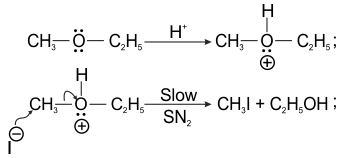General units of Rate constant, relation between half life and initial concentration
Question

# Ethyl methyl ether Products. Correct statements about the reaction are A) It follows ${\mathrm{SN}}^{1}$mechanismB) ${\mathrm{CH}}_{3}\mathrm{I}$ is formedC) ${\mathrm{I}}^{-}$ is the nucleophile

Moderate
Solution

## When a mixed ether containing ${1}^{0}$alkyl group (or) ${\text{2}}^{0}$ alkyl group is heated with conc. HI, the halogen atom always attacks smaller aklyl group. The mechanism followed is ${\mathrm{SN}}^{2}.$Get Instant Solutions